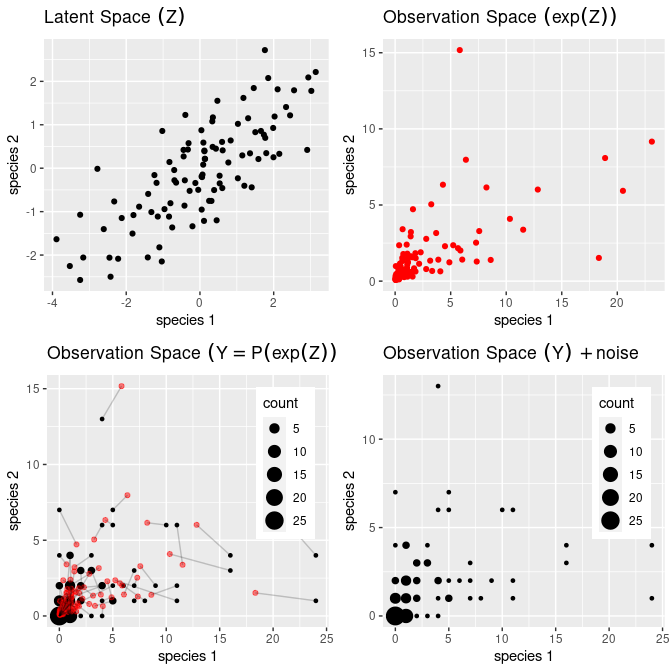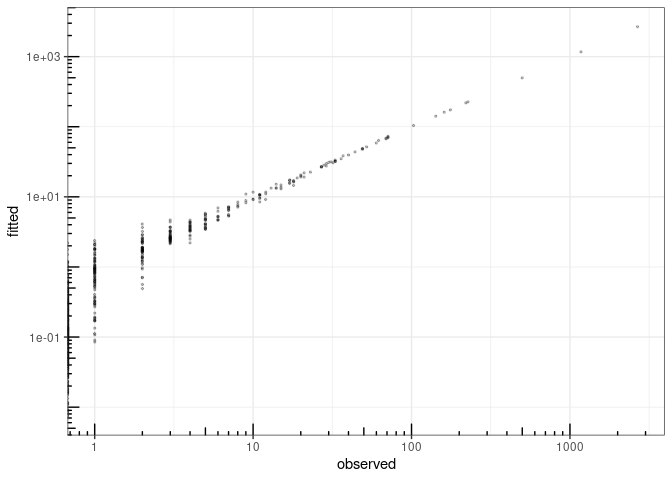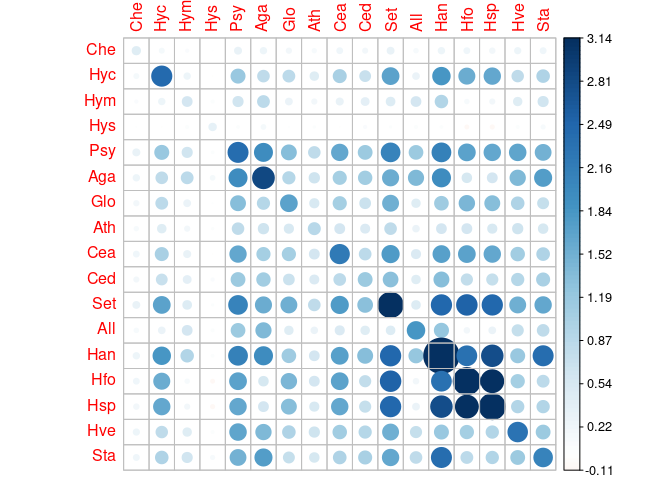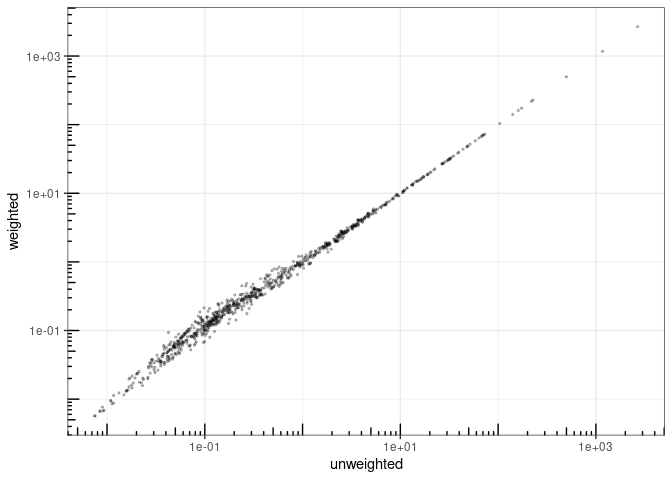# Analyzing multivariate count data with the Poisson log-normal model

## Preliminaries

This vignette illustrates the use of the PLN function and the methods accompanying the R6 class PLNfit.

From the statistical point of view, the function PLN adjusts a multivariate Poisson lognormal model to a table of counts, possibly after correcting for effects of offsets and covariates. PLN is the building block for all the multivariate models found in the PLNmodels package: having a basic understanding of both the mathematical background and the associated set of R functions is a good place to start.

### Requirements

The packages required for the analysis are PLNmodels plus some others for data manipulation and representation:

library(PLNmodels)
library(ggplot2)
library(corrplot)

### Data set

We illustrate our point with the trichoptera data set, a full description of which can be found in the corresponding vignette. Data preparation is also detailed in the specific vignette.

data(trichoptera)
trichoptera <- prepare_data(trichoptera$Abundance, trichoptera$Covariate)

The trichoptera data frame stores a matrix of counts (trichoptera$Abundance), a matrix of offsets (trichoptera$Offset) and some vectors of covariates (trichoptera$Wind, trichoptera$Temperature, etc.)

### Mathematical background

The multivariate Poisson lognormal model (in short PLN, see ) relates some $$p$$-dimensional observation vectors $$\mathbf{Y}_i$$ to some $$p$$-dimensional vectors of Gaussian latent variables $$\mathbf{Z}_i$$ as follows

$\begin{equation} \begin{array}{rcl} \text{latent space } & \mathbf{Z}_i \sim \mathcal{N}({\boldsymbol\mu},\boldsymbol\Sigma), \\ \text{observation space } & Y_{ij} | Z_{ij} \quad \text{indep.} & \mathbf{Y}_i | \mathbf{Z}_i\sim\mathcal{P}\left(\exp\{\mathbf{Z}_i\}\right). \end{array} \end{equation}$

The parameter $${\boldsymbol\mu}$$ corresponds to the main effects and the latent covariance matrix $$\boldsymbol\Sigma$$ describes the underlying residual structure of dependence between the $$p$$ variables. The following figure provides insights about the role played by the different layersPLN: geometrical view

#### Covariates and offsets

This model generalizes naturally to a formulation closer to a multivariate generalized linear model, where the main effect is due to a linear combination of $$d$$ covariates $$\mathbf{x}_i$$ (including a vector of intercepts). We also let the possibility to add some offsets for the $$p$$ variables in in each sample, that is $$\mathbf{o}_i$$. Hence, the previous model generalizes to

$\begin{equation} \mathbf{Y}_i | \mathbf{Z}_i \sim \mathcal{P}\left(\exp\{\mathbf{Z}_i\}\right), \qquad \mathbf{Z}_i \sim \mathcal{N}({\mathbf{o}_i + \mathbf{x}_i^\top\boldsymbol\Theta},\boldsymbol\Sigma), \\ \end{equation}$ where $$\boldsymbol\Theta$$ is a $$d\times p$$ matrix of regression parameters. When all individuals $$i=1,\dots,n$$ are stacked together, the data matrices available to feed the model are

• the $$n\times p$$ matrix of counts $$\mathbf{Y}$$
• the $$n\times d$$ matrix of design $$\mathbf{X}$$
• the $$n\times p$$ matrix of offsets $$\mathbf{O}$$

Inference in PLN then focuses on the regression parameters $$\boldsymbol\Theta$$ and on the covariance matrix $$\boldsymbol\Sigma$$.

#### Optimization by Variational inference

Technically speaking, we adopt in PLNmodels a variational strategy to approximate the log-likelihood function and optimize the consecutive variational surrogate of the log-likelihood with a gradient-ascent-based approach. To this end, we rely on the CCSA algorithm of implemented in the C++ library , which we link to the package.

## Analysis of trichoptera data with a PLN model

The standard PLN model described above is adjusted with the function PLN. We now review its usage on a the trichoptera data set.

### A PLN model with latent main effects

In order to become familiar with the function PLN and its outputs, let us first fit a simple PLN model with just an intercept for each species:

myPLN <- PLN(Abundance ~ 1, trichoptera)
##
##  Initialization...
##  Adjusting a PLN model with full covariance model
##  Post-treatments...
##  DONE!

Note the use of the formula object to specify the model: the vector $$\boldsymbol\mu$$ of main effects in the mathematical formulation (one per column species) is specified in the call with the term ~ 1 in the right-hand-side of the formula. Abundance is a variable in the data frame trichoptera corresponding to a matrix of 17 columns and the response in the model, occurring on the left-hand-side of the formula.

#### The PLNfit object

myPLN is an R6 object with class PLNfit, which comes with a couple of methods, as recalled when printing/showing such an object in the R console:

myPLN
## A multivariate Poisson Lognormal fit with full covariance model.
## ==================================================================
##  nb_param    loglik       BIC       ICL
##       170 -1129.724 -1460.528 -2284.227
## ==================================================================
## * Useful fields
##     $model_par,$latent, $latent_pos,$var_par, $optim_par ##$loglik, $BIC,$ICL, $loglik_vec,$nb_param, $criteria ## * Useful S3 methods ## print(), coef(), sigma(), vcov(), fitted(), predict(), standard_error() See also ?PLNfit for more comprehensive information. #### Field access Accessing public fields of a PLNfit object can be done just like with a traditional list, e.g., c(myPLN$loglik, myPLN$BIC, myPLN$ICL)
##  -1129.724 -1460.528 -2284.227
myPLN$criteria ## nb_param loglik BIC ICL ## 1 170 -1129.724 -1460.528 -2284.227 #### GLM-like interface We provide a set of S3-methods for PLNfit that mimic the standard (G)LM-like interface of R::stats, which we present now. One can access the fitted value of the counts (Abundance$$\hat{\mathbf{Y}}$$) and check that the algorithm basically learned correctly from the data1: data.frame( fitted = as.vector(fitted(myPLN)), observed = as.vector(trichoptera$Abundance)
) %>%
ggplot(aes(x = observed, y = fitted)) +
geom_point(size = .5, alpha =.25 ) +
scale_x_log10() +
scale_y_log10() +
theme_bw() + annotation_logticks()fitted value vs. observation

We can also reach the matrix of regression parameters $$\mathbf{\Theta}$$ and the residual variance/covariance matrix $$\boldsymbol{\Sigma}$$ of the latent variable with the traditional functions found in R for (G)LM manipulation: for the regression coefficients, we can use the coef (or coefficients) method. Approximated standard errors of the coefficients are also accessible via standard_error:

data.frame(
rbind(t(coef(myPLN)), t(standard_error(myPLN))),
row.names = c("effect", "stderr")
) %>% select(1:5) %>% knitr::kable()
Che Hyc Hym Hys Psy
effect -2.9881048 -4.0682558 1.0488723 -2.2685722 3.2711673
stderr 0.5771208 0.5772503 0.0739227 0.4082369 0.0129221

The residual covariance matrix better displays as an image matrix:

corrplot(sigma(myPLN), is.corr = FALSE)#### Observation weights

It is also possible to use observation weights like in standard (G)LMs:

myPLN_weighted <-
PLN(
Abundance ~ 1,
data    = trichoptera,
weights = runif(nrow(trichoptera)),
control = list(trace = 0)
)
data.frame(
unweighted = as.vector(fitted(myPLN)),
weighted   = as.vector(fitted(myPLN_weighted))
) %>%
ggplot(aes(x = unweighted, y = weighted)) +
geom_point(size = .5, alpha =.25 ) +
scale_x_log10() +
scale_y_log10() +
theme_bw() + annotation_logticks()### Accounting for covariates and offsets

For ecological count data, it is generally a good advice to include the sampling effort via an offset term whenever available, otherwise samples are not necessarily comparable:

myPLN_offsets <-
PLN(Abundance ~ 1 + offset(log(Offset)),
data = trichoptera, control = list(trace = 0))

Note that we use the function offset with a log-transform of the total counts since it acts in the latent layer of the model. Obviously the model with offsets is better since the log-likelihood is higher with the same number of parameters2:

rbind(
myPLN$criteria, myPLN_offsets$criteria
) %>% knitr::kable()
nb_param loglik BIC ICL
170 -1129.724 -1460.528 -2284.227
170 -1051.754 -1382.558 -2208.139

Let us try to correct for the wind effect in our model:

myPLN_wind <- PLN(Abundance ~ 1 + Wind + offset(log(Offset)), data = trichoptera)
##
##  Initialization...
##  Adjusting a PLN model with full covariance model
##  Post-treatments...
##  DONE!

When we compare the models, the gain is clear in terms of log-likelihood. However, the BIC chooses not to include this variable:

rbind(
myPLN_offsets$criteria, myPLN_wind$criteria
) %>% knitr::kable()
nb_param loglik BIC ICL
170 -1051.754 -1382.558 -2208.139
187 -1028.085 -1391.970 -2087.008

### Covariance models (full, diagonal, spherical)

It is possible to change a bit the parametrization used for modeling the residual covariance matrix $$\boldsymbol\Sigma$$, and thus reduce the total number of parameters used in the model. By default, the residual covariance is fully parameterized (hence $$p \times (p+1)/2$$ parameters). However, we can chose to only model the variances of the species and not the covariances, by means of a diagonal matrix $$\boldsymbol\Sigma_D$$ with only $$p$$ parameters. In an extreme situation, we may also chose a single variance parameter for the whole matrix $$\boldsymbol\Sigma = \sigma \mathbf{I}_p$$. This can be tuned in PLN with the control argument, a list controlling various aspects of the underlying optimization process:

myPLN_spherical <-
PLN(
Abundance ~ 1 + offset(log(Offset)),
data = trichoptera, control = list(covariance = "spherical", trace = 0)
)
myPLN_diagonal <-
PLN(
Abundance ~ 1 + offset(log(Offset)),
data = trichoptera, control = list(covariance = "diagonal", trace = 0)
)

Note that, by default, the model chosen is covariance = "spherical", so that the two following calls are equivalents:

myPLN_default <-
PLN(Abundance ~ 1, data = trichoptera, )
##
##  Initialization...
##  Adjusting a PLN model with full covariance model
##  Post-treatments...
##  DONE!
myPLN_full <-
PLN(Abundance ~ 1, data = trichoptera, control = list(covariance = "full"))
##
##  Initialization...
##  Adjusting a PLN model with full covariance model
##  Post-treatments...
##  DONE!

Different covariance models can then be compared with the usual criteria: it seems that the gain brought by passing from a diagonal matrix to a fully parameterized covariance is not worth having so many additional parameters:

rbind(
myPLN_offsets$criteria, myPLN_diagonal$criteria,
myPLN_spherical$criteria ) %>% as.data.frame(row.names = c("full", "diagonal", "spherical")) %>% knitr::kable() nb_param loglik BIC ICL full 170 -1051.754 -1382.558 -2208.139 diagonal 34 -1109.691 -1175.852 -2080.825 spherical 18 -1398.710 -1433.736 -1891.430 A final model that we can try is the diagonal one with the wind as a covariate, which gives a slight improvement. myPLN_final <- PLN( Abundance ~ 1 + Wind + offset(log(Offset)), data = trichoptera, control = list(covariance = "diagonal", trace = 0) ) rbind( myPLN_wind$criteria,
myPLN_diagonal$criteria, myPLN_final$criteria
) %>% knitr::kable()
nb_param loglik BIC ICL
187 -1028.085 -1391.970 -2087.008
34 -1109.691 -1175.852 -2080.825
51 -1073.053 -1172.295 -1750.145

1. We use a log-log scale in our plot in order not to give an excessive importance to the higher counts in the fit↩︎

2. In PLNmodels the R-squared is a pseudo-R-squared that can only be trusted between model where the same offsets term was used↩︎